# 手把手教你 SQL 多表查询

- 学生表：Student（ Sno，Sname，Ssex，Sage，Sdept ）
- 课程表：Course（ Cno，Cname，Cpno，Ccredit）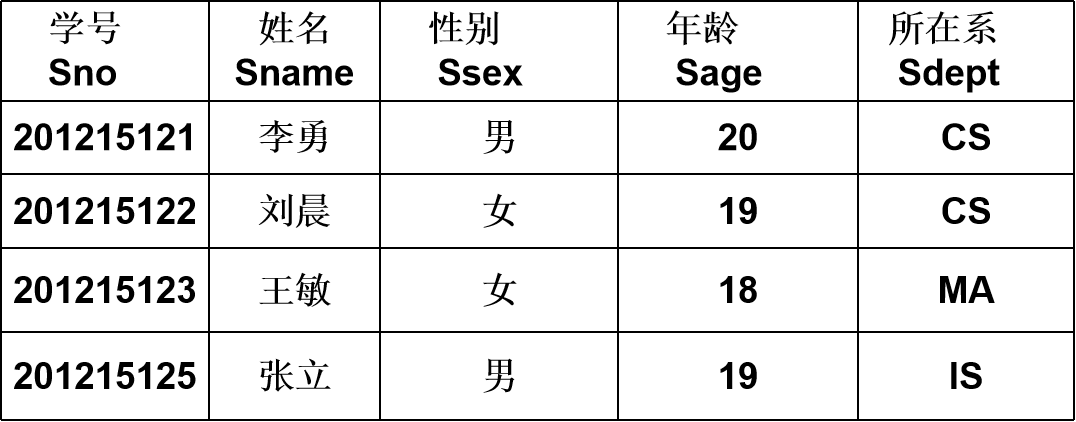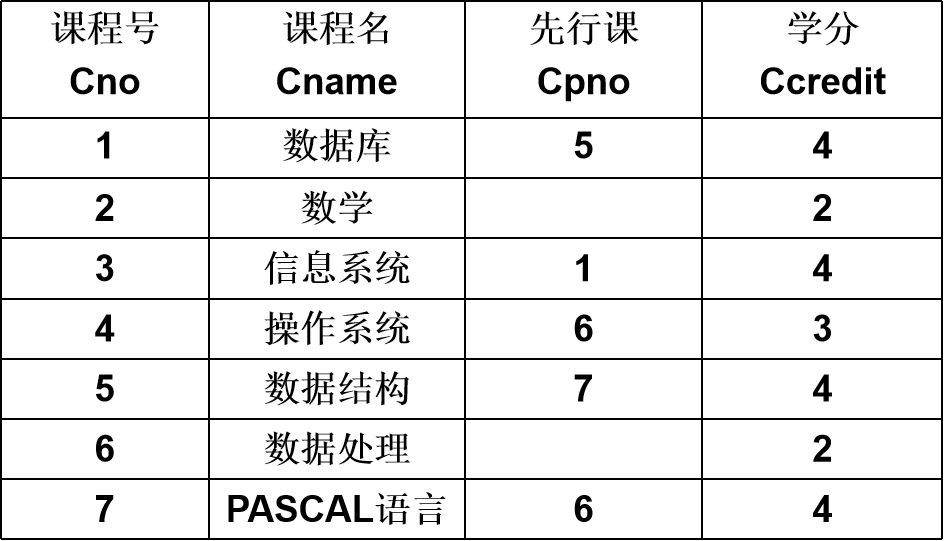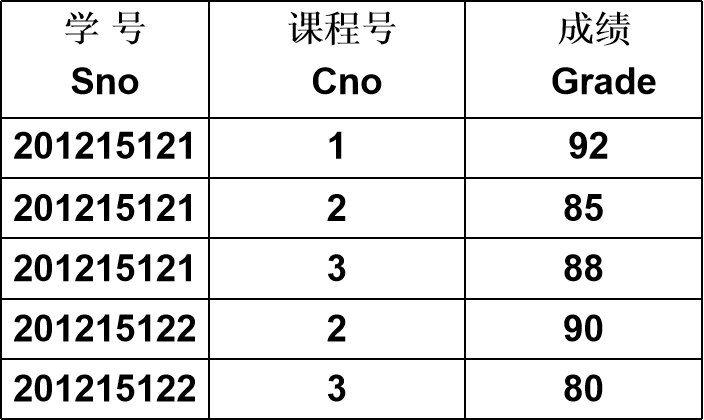## 一、连接查询

### 1、等值与非等值连接查询

SELECT Student.*, SC.*
FROM Student,
SC
WHERE Student.Sno = SC.Sno;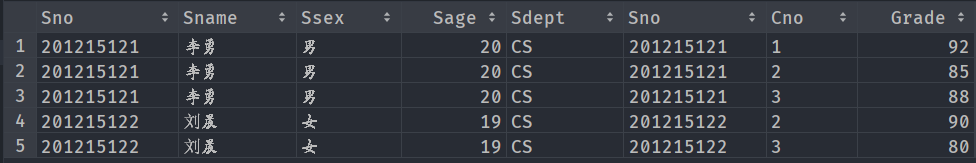# 使用内连接
select *
from student
inner join
sc
on student.Sno = sc.Sno;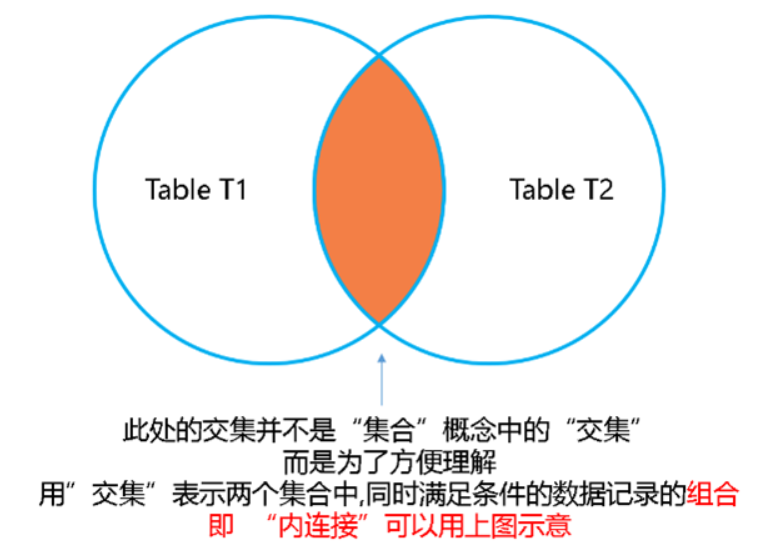另外在 MySQL 中，inner join 可以简写成 join


• 首先在表 1 中找到第一个元组，然后从头开始扫描表 2 ，逐一查找满足连接件的元组，找到后就将表1中的第一个元组与该元组拼接起来，形成结果表中一个元组。
• 表 2 全部查找完后，再找表1中第二个元组，然后再从头开始扫描表 2 ，逐一查找满足连接条件的元组，找到后就将表 1 中的第二个元组与该元组拼接起来，形成结果表中一个元组。
• 重复上述操作，直到表 1 中的全部元组都处理完毕

• 常用于 = 连接
• 首先按连接属性对表 1 和 2 排序
• 对表 1 的第一个元组，从头开始扫描表 2 ，顺序查找满足连接条件的元组，找到后就将表 1 中的第一个元组与该元组拼接起来，形成结果表中一个元组。当遇到表 2 中第一条大于表 1 连接字段值的元组时，对表 2 的查询不再继续
• 找到表 1 的第二条元组，然后从刚才的中断点处继续顺序扫描表 2 ，查找满足连接条件的元组，找到后就将表 1 中的第一个元组与该元组拼接起来，形成结果表中一个元组。直接遇到表 2 中大于表 1 连接字段值的元组时，对表 2 的查询不再继续
• 重复上述操作，直到表 1 或表 2 中的全部元组都处理完毕为止

• 对表2按连接字段建立索引
• 对表1中的每个元组，依次根据其连接字段值查询表2的索引，从中找到满足条件的元组，找到后就将表1中的第一个元组与该元组拼接起来，形成结果表中一个元组

• 先从SC中挑选出Cno='2'并且Grade>90的元组形成一个中间关系；
• 再和Student中满足连接条件的元组进行连接得到最终的结果关系；

### 2、自身连接

• 自身连接：一个表与其自己进行连接
• 需要给表起别名以示区别
• 由于所有属性名都是同名属性，因此必须使用别名前缀

select c1.Cno first, c2.Cpno second
from course c1, course c2
where c1.Cpno = c2.Cno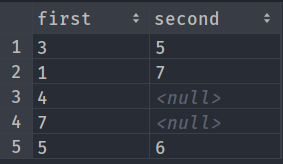### 3、外连接

• 普通连接操作只输出满足连接条件的元组
• 外连接操作以指定表为连接主体，将主体表中不满足连接条件的元组一并输出
• 左外连接：列出左边关系中所有的元组
• 右外连接：列出右边关系中所有的元组

SELECT Student.Sno, Sname, Ssex, Sage, Sdept, Cno, Grade
FROM Student
LEFT OUTER JOIN SC
ON
(Student.Sno = SC.Sno);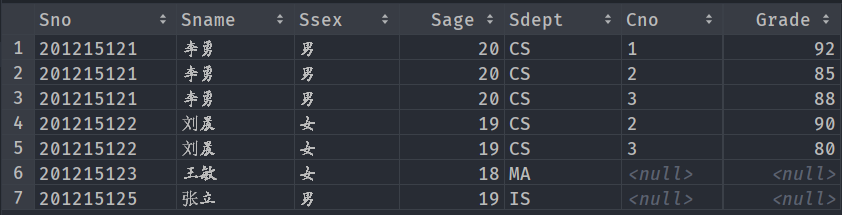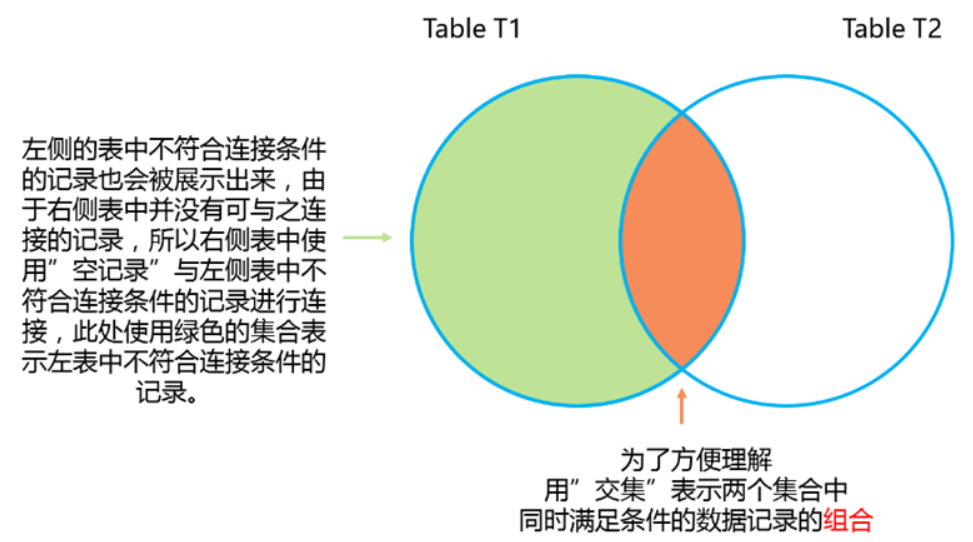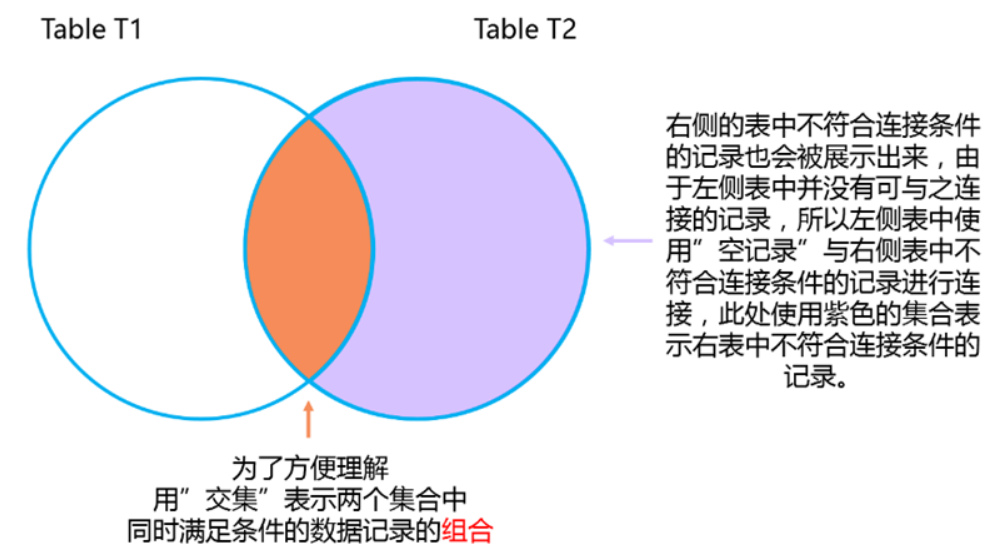left outer join 可以简写成 left join


select *
from student
left join sc
on student.Sno = sc.Sno
where Cno = 3;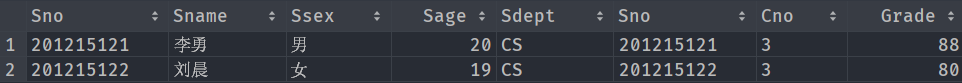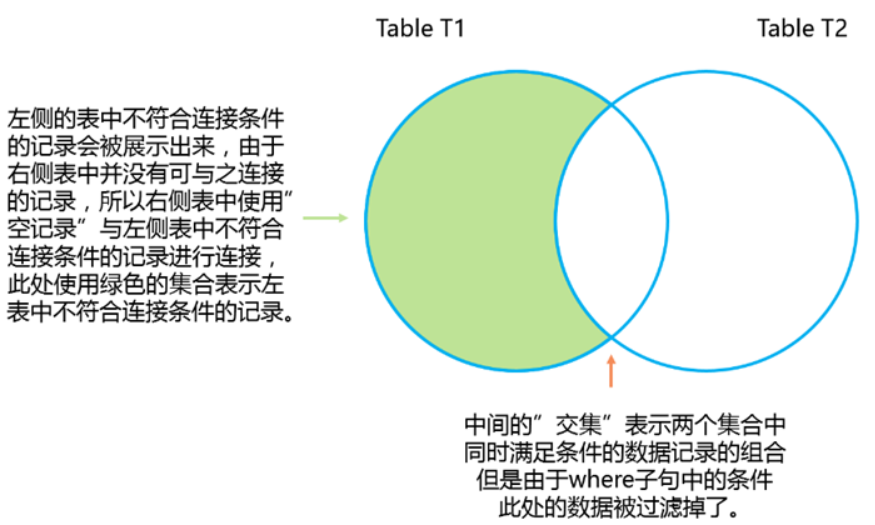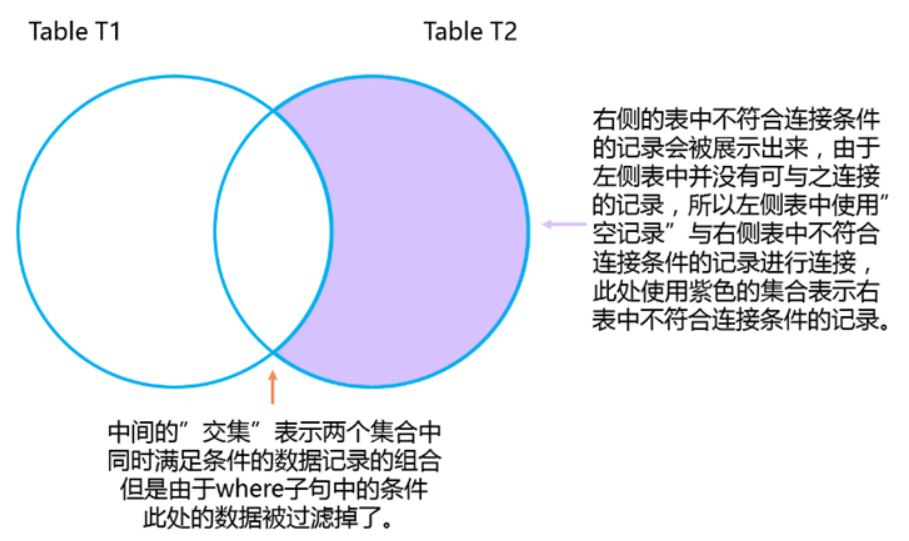### 4、多表连接

SELECT Student.Sno, Sname, Cname, Grade
FROM Student,
SC,
Course /*多表连接*/
WHERE Student.Sno = SC.Sno
AND SC.Cno = Course.Cno;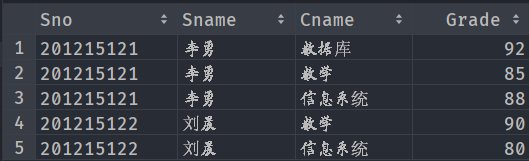## 二、嵌套查询

• 一个SELECT-FROM-WHERE语句称为一个查询块
• 将一个查询块嵌套在另一个查询块的WHERE子句或HAVING短语的条件中的查询称为嵌套查询
SELECT Sname /*外层查询/父查询*/
FROM Student
WHERE Sno IN
(SELECT Sno /*内层查询/子查询*/
FROM SC
WHERE Cno = ' 2 ');

• 上层的查询块称为外层查询或父查询
• 下层查询块称为内层查询或子查询
• SQL语言允许多层嵌套查询
• 即一个子查询中还可以嵌套其他子查询
• 子查询的限制
• 不能使用ORDER BY子句

### 1、带有IN谓词的子查询

① 确定“刘晨”所在系名：

SELECT Sdept
FROM Student
WHERE Sname = '刘晨';


② 查找所有在CS系学习的学生。

SELECT Sno, Sname, Sdept
FROM Student
WHERE Sdept = 'CS';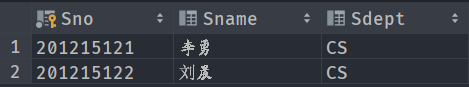SELECT Sno, Sname, Sdept
FROM Student
WHERE Sdept IN
(SELECT Sdept
FROM Student
WHERE Sname = '刘晨');


SELECT S1.Sno, S1.Sname, S1.Sdept
FROM Student S1,
Student S2
WHERE S1.Sdept = S2.Sdept
AND S2.Sname = '刘晨';


select Sno, Sname
from student
where Sno in (
select sc.Sno
from sc
where Cno in (
select course.Cno
from course
where Cname = '信息系统'
)
)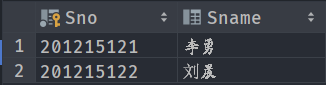# 使用连接查询实现上述功能
select student.Sno, Sname
from student,
sc,
course
where student.Sno = sc.Sno
and sc.Cno = course.Cno
and Cname = '信息系统';


### 2、带有比较运算符的子查询

• 当能确切知道内层查询返回一个值时，可用比较运算符；
SELECT Sno, Sname, Sdept
FROM Student
WHERE Sdept =
(SELECT Sdept
FROM Student
WHERE Sname = '刘晨');


# 找出每个学生超过他选修课程平均成绩的课程号。
select x.Sno, x.Cno
from sc x
from sc y
where x.Sno = y.Sno
);


• 从外层查询中取出 SC 的一个元组 x ，将元组 x 的 Sno 值（201215121）传送给内层查询。
SELECT AVG(Grade)
FROM SC y
WHERE y.Sno='201215121';

• 执行内层查询，得到值88（近似值），用该值代替内层查询，得到外层查询：
SELECT Sno,Cno
FROM   SC x

• 执行这个查询，得到
（201215121,1）
（201215121,3）

• 然后外层查询取出下一个元组重复做上述①至③步骤，直到外层的SC元组全部处理完毕。结果为:
（201215121,1）
（201215121,3）
（201215122,2）


### 3、带有 ANY（SOME）或ALL 谓词的子查询

> ANY大于子查询结果中的某个值
> ALL大于子查询结果中的所有值
< ANY小于子查询结果中的某个值
< ALL小于子查询结果中的所有值
>= ANY大于等于子查询结果中的某个值
>= ALL大于等于子查询结果中的所有值
<= ANY小于等于子查询结果中的某个值
<= ALL小于等于子查询结果中的所有值
= ANY等于子查询结果中的某个值
=ALL等于子查询结果中的所有值（通常没有实际意义）
!=（或<>）ANY不等于子查询结果中的某个值
!=（或<>）ALL不等于子查询结果中的任何一个值

SELECT Sname, Sage
FROM Student
WHERE Sage < ANY (SELECT Sage
FROM Student
WHERE Sdept = 'CS')
AND Sdept <> 'CS';


• 首先处理子查询，找出 CS 系中所有学生的年龄，构成一个集合（20,19）
• 处理父查询，找所有不是 CS 系且年龄小于 20 或 19的学生

# 用聚集函数实现
SELECT Sname, Sage
FROM Student
WHERE Sage <
(SELECT MAX(Sage)
FROM Student
WHERE Sdept = 'CS')
AND Sdept <> 'CS';


# 查询非计算机科学系中比计算机科学系所有学生年龄都小的学生姓名及年龄。
select student.Sname, student.Sage
from student
where Sage < all (
select Sage
from student
where Sdept = 'CS'
)
and Sdept <> 'CS';


# 使用聚合函数
select student.Sname, student.Sage
from student
where Sage < (
select min(sage)
from student
where Sdept = 'CS'
)
and Sdept <> 'CS';


### 4、带有 EXISTS 谓词的子查询

• exists 是存在量词 ∃ \exist .
• 带有 EXISTS 谓词的子查询不返回任何数据，只产生逻辑真值 “true” 或逻辑假值 “false” 。

• 本查询涉及 Student 和 SC 关系
• 在 Student 中依次取每个元组的 Sno 值，用此值去检查 SC 表
• 若 SC 中存在这样的元组，其 Sno 值等于此 Student.Sno 值，并且其 Cno= ‘1’ ，则取此Student.Sname 送入结果表
# 查询所有选修了1号课程的学生姓名。
select Sname
from student
where exists(
select *
from sc
where sc.Sno = student.Sno
and Cno = '1');


select Sname
from student
where Sno in (select sc.Sno
from sc
where Cno = '1');


select Sname
from student
where not exists(
select *
from sc
where Cno = '1'
and sc.Sno = student.Sno
);


• 一些带 EXISTS 或 NOT EXISTS 谓词的子查询不能被其他形式的子查询等价替换
• 所有带 IN 谓词、比较运算符、ANY 和 ALL 谓词的子查询都能用带 EXISTS 谓词的子查询等价替换

## 三、集合查询

• 并操作 UNION
• 交操作 INTERSECT
• 差操作 EXCEPT

SELECT *
FROM Student
WHERE Sdept = 'CS'
UNION
SELECT *
FROM Student
WHERE Sage <= 19;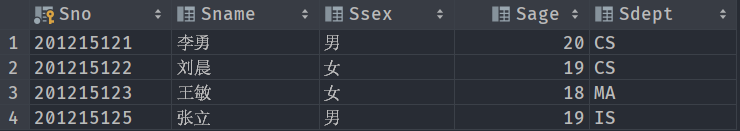UNION：将多个查询结果合并起来时，系统自动去掉重复元组；
UNION ALL：将多个查询结果合并起来时，保留重复元组。

SELECT Sno
FROM SC
WHERE Cno = '1'
UNION
SELECT Sno
FROM SC
WHERE Cno = '2';


SELECT *
FROM Student
WHERE Sdept = 'CS'
AND Sage <= 19;


## 四、源码

# 查询每个学生及其选修课程的情况
SELECT Student.*, SC.*
FROM Student,
SC
WHERE Student.Sno = SC.Sno;

# 使用内连接
select *
from student
inner join
sc
on student.Sno = sc.Sno;

# 查询选修2号课程且成绩在90分以上的所有学生的学号和姓名。
SELECT Student.Sno, Sname
FROM Student,
SC
WHERE Student.Sno = SC.Sno
AND SC.Cno = '2'

# 查询每一门课的间接先修课（即先修课的先修课）
select c1.Cno first, c2.Cpno second
from course c1,
course c2
where c1.Cpno = c2.Cno;

SELECT Student.Sno, Sname, Ssex, Sage, Sdept, Cno, Grade
FROM Student
LEFT OUTER JOIN SC
ON
(Student.Sno = SC.Sno);

select *
from student
left join sc
on student.Sno = sc.Sno
where Cno = 3;

# 查询每个学生的学号、姓名、选修的课程名及成绩
FROM Student,
SC,
Course /*多表连接*/
WHERE Student.Sno = SC.Sno
AND SC.Cno = Course.Cno;

SELECT Sname /*外层查询/父查询*/
FROM Student
WHERE Sno IN
(SELECT Sno /*内层查询/子查询*/
FROM SC
WHERE Cno = ' 2 ');

# 查询与“刘晨”在同一个系学习的学生 的3种实现方式
# 第一种
SELECT Sdept
FROM Student
WHERE Sname = '刘晨';

SELECT Sno, Sname, Sdept
FROM Student
WHERE Sdept = 'CS';

# 第二种
SELECT Sno, Sname, Sdept
FROM Student
WHERE Sdept =
(SELECT Sdept
FROM Student
WHERE Sname = '刘晨');

# 第三种
SELECT S1.Sno, S1.Sname, S1.Sdept
FROM Student S1,
Student S2
WHERE S1.Sdept = S2.Sdept
AND S2.Sname = '刘晨';

# 查询选修了课程名为“信息系统”的学生学号和姓名
select Sno, Sname
from student
where Sno in (
select sc.Sno
from sc
where Cno in (
select course.Cno
from course
where Cname = '信息系统'
)
);

# 使用连接查询实现上述功能
select student.Sno, Sname
from student,
sc,
course
where student.Sno = sc.Sno
and sc.Cno = course.Cno
and Cname = '信息系统';

SELECT *
FROM Student
WHERE Sdept = 'CS'
UNION
SELECT *
FROM Student
WHERE Sage <= 19;

SELECT Sno
FROM SC
WHERE Cno = '1'
UNION
SELECT Sno
FROM SC
WHERE Cno = '2';

# 找出每个学生超过他选修课程平均成绩的课程号。
select x.Sno, x.Cno
from sc x
from sc y
where x.Sno = y.Sno
);

# 查询非计算机科学系中比计算机科学系任意一个学生年龄小的学生姓名和年龄。
SELECT Sname, Sage
FROM Student
WHERE Sage < ANY (SELECT Sage
FROM Student
WHERE Sdept = 'CS')
AND Sdept <> 'CS';

# 用聚集函数实现
SELECT Sname, Sage
FROM Student
WHERE Sage <
(SELECT MAX(Sage)
FROM Student
WHERE Sdept = 'CS')
AND Sdept <> 'CS';

# 查询非计算机科学系中比计算机科学系所有学生年龄都小的学生姓名及年龄。
select student.Sname, student.Sage
from student
where Sage < all (
select Sage
from student
where Sdept = 'CS'
)
and Sdept <> 'CS';

# 使用聚合函数
select student.Sname, student.Sage
from student
where Sage < (
select min(sage)
from student
where Sdept = 'CS'
)
and Sdept <> 'CS';

# 查询所有选修了1号课程的学生姓名。
select Sname
from student
where exists(
select *
from sc
where sc.Sno = student.Sno
and Cno = '1');

# 使用谓词IN实现
select Sname
from student
where Sno in (select sc.Sno
from sc
where Cno = '1');

# 查询没有选修1号课程的学生姓名。
select Sname
from student
where not exists(
select *
from sc
where Cno = '1'
and sc.Sno = student.Sno
);

SELECT *
FROM Student
WHERE Sdept = 'CS'
AND Sage <= 19;

SELECT Sno
FROM SC
WHERE Cno = '1'
and Cno = '2'
;

SELECT Sno
FROM SC
WHERE Cno = '1'
AND Sno IN
(SELECT Sno
FROM SC
WHERE Cno = '2');

06-051万+

05-292923
11-273322
08-08965
06-122677
06-242849
01-1611万+
03-162809
11-061977
01-254914
11-24979
12-037449
11-271万+
08-02666
05-06365
10-21199

### “相关推荐”对你有帮助么？

•非常没帮助
•没帮助
•一般
•有帮助
•非常有帮助¥2 ¥4 ¥6 ¥10 ¥20余额支付 (余额：-- )扫码支付获取中扫码支付点击重新获取扫码支付1.余额是钱包充值的虚拟货币，按照1:1的比例进行支付金额的抵扣。
2.余额无法直接购买下载，可以购买VIP、C币套餐、付费专栏及课程。余额充值